UPSC  >  Arithmetic Progression: Solved Examples

# Arithmetic Progression: Solved Examples - CSAT Preparation - UPSC

Sequence & Series

A set of numbers whose domain is a real number is called a SEQUENCE and sum of the sequence is called a
SERIES. If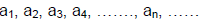is a sequence, then the expression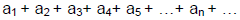is
a series.
Those sequences whose terms follow certain patterns are called progressions.

For example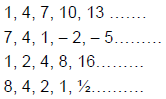Also if f (n) = n2 is a sequence, then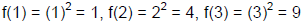f (10) = 102 = 100 and so on.

The nth term of a sequence is usually denoted by Tn
Thus T1 = first term, T2 = second term, T10 = tenth term and so on.

There are three different progressions

1. Arithmetic Progression (A.P)
2. Geometric Progression (G.P)
3. Harmonic Progression (H.P)

Arithmetic Progression
It is a series in which any two consecutive terms have common difference and next term can be derived by
adding that common difference in the previous term.
Therefore Tn+1 - Tn = constant and called common difference (d) for all n ∈ N.

Examples:

1. 1, 4, 7, 10, ……. is an A. P. whose first term is 1 and the common difference is
d = (4 - 1) = (7 - 4) = (10 - 7) = 3.

2. 11, 7, 3, - 1 …… is an A. P. whose first term is 11 and the common difference
d = 7 - 11 = 3 - 7, = - 1 - 3 = - 4.

If in an A. P.

a = first term,
d = common difference = Tn - Tn-1
Tn = nth term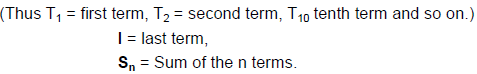Then a, a + d, a + 2d, a + 3d,... are in A.P.
nth term of an A.P.

The nth term of an A.P is given by the formula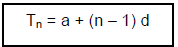Note: If the last term of the A.P. consisting of n terms be l , then
l = a + (n - 1) d

Sum of n terms of an A.P
The sum of first n terms of an AP is usually denoted by Sn and is given by the following formula: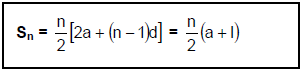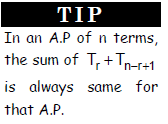Where ‘l ’ is the last term of the series.

Ex.1 Find the series whose nth term is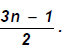. Is it an A. P. series? If yes, find 101st term.

Sol. Putting 1, 2, 3, 4…. We get T1, T2, T3, T4…………..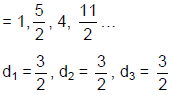As the common differences are equal
∴The series is an A.P.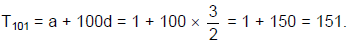Ex.2 Find 8th, 12th and 16th terms of the series; - 6, - 2, 2, 6, 10, 14, 18…

Sol.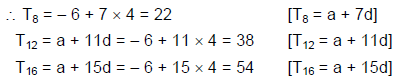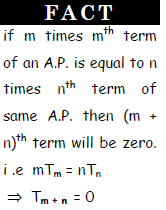Properties of an AP
I. If each term of an AP is increased, decreased, multiplied or divided by the
same non-zero number, then the resulting sequence is also an AP.

For example: For A.P. 3, 5, 7, 9, 11…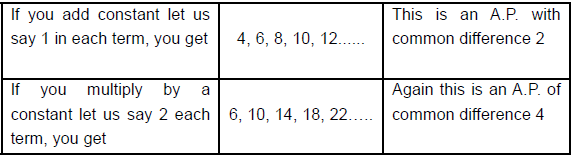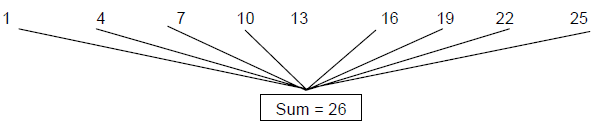II. In an AP, the sum of terms equidistant from the beginning and end is always same and equal to the sum
of first and last terms as shown in example below.

III. Three numbers in AP are taken as a - d, a, a + d.
For 4 numbers in AP are taken as a - 3d, a - d, a + d, a + 3d.
For 5 numbers in AP are taken as a - 2d, a - d, a, a + d, a + 2d.

IV. Three numbers a, b, c are in A.P. if and only if
2b = a + c.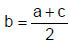and b is called Arithmetic mean of a & c

Ex.3 The sum of three numbers in A.P. is - 3, and their product is 8. Find the numbers.

Sol. Let the numbers be (a - d), a, (a + d). Then,
Sum = - 3 ⇒ (a - d) + a + (a + d) = - 3 ⇒ 3a = - 3 ⇒ a = - 1
Product = 8
⇒ (a - d) (a) (a + d) = 8
⇒ a (a2 - d2) = 8
⇒ (-1) (1 - d2) = 8
⇒ d2 = 9
⇒ d = ± 3
If d = 3, the numbers are - 4, - 1, 2. If d = - 3, the numbers are 2, - 1, - 4.
Thus, the numbers are - 4, - 1, 2 or 2, - 1, - 4.

Ex.4 A student purchases a pen for Rs. 100. At the end of 8 years, it is valued at Rs. 20. Assuming
that the yearly depreciation is constant. Find the annual depreciation.

Sol. Original cost of pen = Rs. 100
Let D be the annual depreciation.
∴ Price after one year = 100 - D = T1 = a (say)
∴ Price after eight years = T8 = a + 7 (- D) = a - 7D
= 100 - D - 7D = 100 - 8D
By the given condition 100 - 8D = 20
8D = 80

∴D = 10.
Hence annual depreciation = Rs. 10.

The document Arithmetic Progression: Solved Examples | CSAT Preparation - UPSC is a part of the UPSC Course CSAT Preparation.
All you need of UPSC at this link: UPSC

## CSAT Preparation

197 videos|151 docs|200 tests

## FAQs on Arithmetic Progression: Solved Examples - CSAT Preparation - UPSC

 1. What is an arithmetic progression?An arithmetic progression is a sequence of numbers in which the difference between any two consecutive terms is constant. This difference is called the common difference, denoted by 'd'.
 2. How can I find the nth term of an arithmetic progression?To find the nth term of an arithmetic progression, you can use the formula: nth term = a + (n-1)d, where 'a' is the first term and 'd' is the common difference. Simply substitute the values of 'a', 'n', and 'd' into the formula to get the desired term.
 3. Can an arithmetic progression have a negative common difference?Yes, an arithmetic progression can have a negative common difference. The common difference can be positive, negative, or zero, depending on the sequence. For example, an arithmetic progression with a common difference of -2 would be: 10, 8, 6, 4, ...
 4. How can I find the sum of an arithmetic progression?To find the sum of an arithmetic progression, you can use the formula: sum = (n/2)(2a + (n-1)d), where 'n' is the number of terms, 'a' is the first term, and 'd' is the common difference. Substitute the values of 'n', 'a', and 'd' into the formula to calculate the sum.
 5. Is it possible for an arithmetic progression to have an infinite number of terms?No, an arithmetic progression cannot have an infinite number of terms. Since the difference between consecutive terms is constant, the progression will eventually reach a point where it does not continue indefinitely. However, the number of terms can be very large depending on the context.

## CSAT Preparation

197 videos|151 docs|200 testsExplore Courses for UPSC exam### How to Prepare for UPSC

Read our guide to prepare for UPSC which is created by Toppers & the best Teachers
Signup to see your scores go up within 7 days! Learn & Practice with 1000+ FREE Notes, Videos & Tests.
10M+ students study on EduRev
Track your progress, build streaks, highlight & save important lessons and more!
Related Searches

,

,

,

,

,

,

,

,

,

,

,

,

,

,

,

,

,

,

,

,

,

;